﻿

### The submanifolds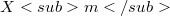$Xm$ of the manifold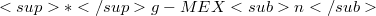$*g-MEXn$. I. The induced connection on$Xm$ of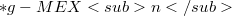$*g-MEXn$

#### Abstract

An Einstein's connection which takes the form (2.33) is called an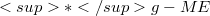$*g-ME$-connection. Recently, Chung and et al (, 1993)introduced a new manifolds, called an n-dimensional$*g-ME$-manifold (debnoted by$*g-MEXn$).The manifold$*g-MEXn$ is a generalized n-dimensional Riemannian manifold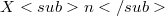$Xn$ on which the differential geometric structure is imposed by the unified field tensor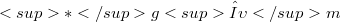$*gλ \upsilon m$ satisfying certain conditions through the$*g-ME$-connection. In the following series of two papers, we investigate the submanifolds$Xm$ of$*g-MEXn$: I. The induced connection on$Xm$ of$*g-MEXn$ II. The generalized fundamental equations on$Xm$ of$*g-MEXn$ In this paper, Part I of the series, we present a brief introduction of n-dimensional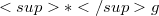$*g$-unified field theory, the C-nonholonomic frame of reference in$Xn$ at points of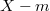$X-m$, and the manifold$*g-MEXn$. and then, we introduce the generalized coefficients of the second fundamental form of$Xm$ and prove a necessary and sufficient condition for the induced connection on$Xm$ of$*-MEXn$ to be a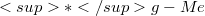$*g-Me$-connection. Our subsequent paper, Part II of the series, deals with the generalized fundamental equations on$Xm$ of$*-MEXn$, such as the generalized Gauss formulae, the generalized Weingarten equations, and the Gauss-Codazzi equations.

DOI Code: 10.1285/i15900932v18n2p213

Full Text: PDF